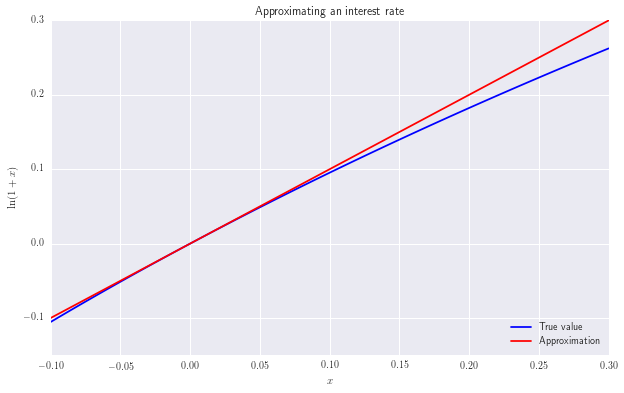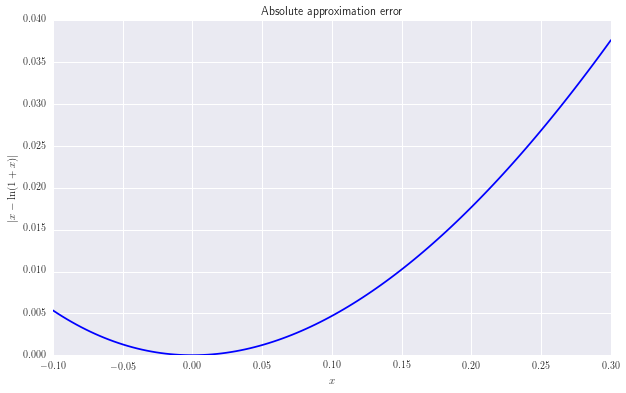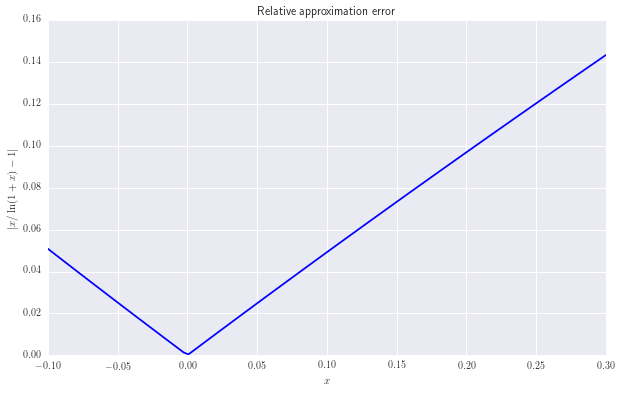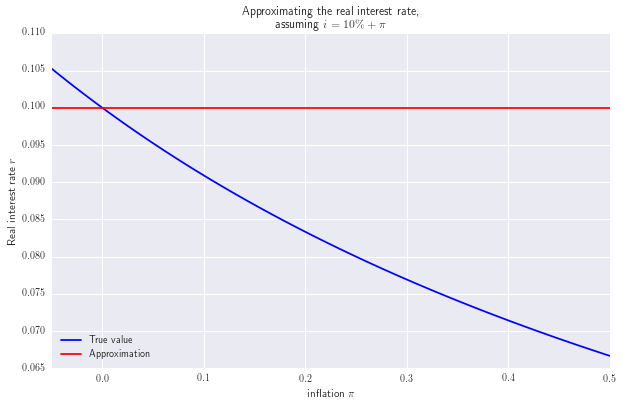# Approximating real interest rates¶

Randall Romero Aguilar

August 2016

The real interest rate $r$ is defined in terms of the nominal interest rate $i$ and the inflation rate $\pi$ by \begin{equation} 1+r = \frac{1+i}{1+\pi} \end{equation} which is usually approximated by \begin{equation} r \approx i - \pi \end{equation}

## Why it works¶

• Taylor approximation

This approximation is based on the first-order Taylor approximation of $\ln(1+x) \approx x$, which is fairly accurate for small values of $x$. Thus, using Taylor approximation we see that the real interest rate is \begin{align} 1+r &= \frac{1+i}{1+\pi} \\\\ \ln(1+r) &= \ln(1+i) - \ln(1+\pi) \\\\ r &\approx i - \pi \end{align}

• An easier way

From the definition of the real interest rate, \begin{align} (1+r)(1+\pi) &= 1+i \\\\ 1+r+\pi+r\pi &= 1+i \\\\ r &= i - \pi - r\pi \\\\ r &\approx i - \pi \end{align} where the last step follows because $r\pi$ is a small quantity as long as $r$ and $\pi$ are small.

This numerical example illustrates the magnitude of approximation errors that we induce by taking this approximation.

### Set up¶

• Import necessary libraries
In :
import numpy as np
import matplotlib.pyplot as plt
import seaborn
%matplotlib inline
plt.rc('text', usetex=True)
plt.rcParams['figure.figsize'] = 10, 6

• Generate data
In :
a, b = -0.1, 0.3
x = np.linspace(a, b, 120)
y = np.log(1 + x)
absolute_error = np.abs(x - y)
relative_error = np.abs(x / y - 1)

• This utility function we'll make it easier to work with the plots below
In :
def common_options(title, xlab, ylab):
plt.xlim([a, b])
plt.xlabel(xlab)
plt.ylabel(ylab)
plt.title(title)
return


### Plots¶

• The function and its approximation
In :
plt.plot(x, y, 'b-', label='True value')
plt.plot(x, x, 'r-', label='Approximation')
common_options('Approximating an interest rate','$x$', '$\ln(1 + x)$')
plt.legend(loc='lower right', frameon=False)

Out:
<matplotlib.legend.Legend at 0x25390ed6f60>• Absolute errors

Notice that the approximation error is less than half a percentage point as long as $x$ is less than 10% in absolute value.Beyond that range, the error grows quickly

In :
plt.plot(x, absolute_error, 'b-')
common_options('Absolute approximation error', '$x$', '$| x - \ln(1 + x) |$')• Relative errors

Here what we are interested in the size of the approximation error relative to the original interest rate. For example, for a 20% interest rate, interest payments computed with the appoximated formula would be off by 10 percent.

In :
plt.plot(x, relative_error, 'b-')
common_options('Relative approximation error', '$x$', '$| x / \ln(1 + x) - 1|$')### Constant difference between nominal interest and inflation¶

In this example, we assume that the nominal interest rate is always 10percentage points above the inflation rate. Notice that in such case, for high inflation rates the approximation deteriorates quickly.

In :
a, b = -0.05, 0.50
inflation = np.linspace(a, b, 121)
nominal = inflation + 0.10
r = (1+nominal) / (1+inflation) - 1

plt.plot(inflation, r, 'b-', label='True value')
plt.plot(inflation, 0.10+np.zeros_like(inflation), 'r-', label='Approximation')
common_options('Approximating the real interest rate,\nassuming $i=10\%+\pi$', 'inflation $\pi$', 'Real interest rate $r$')
plt.legend(loc='lower left', frameon=False)

Out:
<matplotlib.legend.Legend at 0x25390f5cf28>